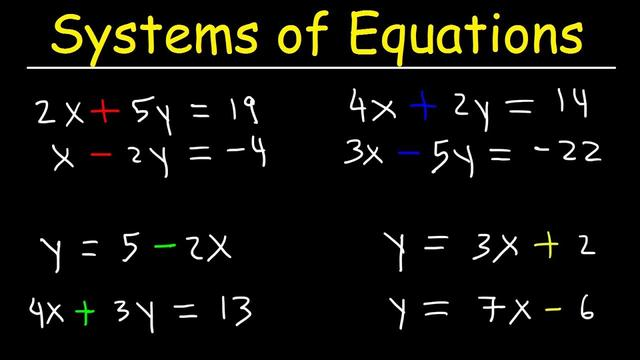Home Technology System of equations

# System of equations

## Definition

A system of equations, also known as simultaneous equations, is a combination of two or more equations containing multiple unknowns. The value of the unknown is called the "solutions" of the system of equations, and the process of finding the roots of the system of equations is called "solving the system of equations". Generally, it is marked in enlarged brackets on the left side of the equation.

Generally start to learn two-variable linear equations or three-variable linear equations at the junior high school stage.

The combination of two or more equations is called a system of equations.

The overall idea of ​​solving equations is elimination, which includes addition and subtraction elimination method and substitution elimination method.

## Methods of solving equations

The methods of solving equations generally include drawing method, matrix method, substitution method, elimination method and so on.

### 1. Substitution method

To solve the following equations:, the solution process of substitution method is:

Then substitute it into one of the equations:.

So its solution is:.### 2. The drawing method

The drawing method is to draw two equations on the graph, and the intersection of the two lines is the solution. To solve the following equations:

First, we must draw them on the graph:

The green is, the red is.

The intersection of the two lines is their solution:.

### 3. Elimination method

If you want to use the elimination method to solve the following equations:.

Subtract the left and right sides of the equal sign of the two equations separately: the above formula-the following formula,

Then substitute it into one of the equations:

Get:.

## Examples

Example. 4 baskets of apples and 3 baskets of pears weigh 310 kg, 3 baskets of apples and 5 baskets of pears weigh 370 kg. How many kilograms each basket of apples and pears weigh ?

Analysis and answer: Let’s say that apples are kilograms per basket, and pears are kilograms per basket. According to the 4 baskets of apples and 3 baskets of pears weighing 310 kg, formulate the equation; and then the 3 baskets of apples and 5 baskets of pears weighing 370 kg to formulate the equation

②< /p>

Multiply both sides of the formula by 3, get ③

Multiply both sides of the formula by 4, get ④

Subtract formula ③ from formula ④, get < /p>